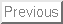# Convergence of density-matrix expansions for nuclear interactions

B.G. Carlsson and J. Dobaczewski

July 28, 2010

### Abstract:

We extend density-matrix expansions in nuclei to higher orders in derivatives of densities and test their convergence properties. The expansions allow for converting the interaction energies characteristic to finite- and short-range nuclear effective forces into quasi-local density functionals. We also propose a new type of expansion that has excellent convergence properties when benchmarked against the binding energies obtained for the Gogny interaction.

The description of finite many-body systems is a continuous exciting challenge in physics. In view of the exploding dimensionality of many-particle Hilbert spaces, direct approaches are however often untractable. Instead, density functional theory (DFT)  provides an alternative by showing that a much simpler description in terms of the density alone is in principle possible. The main challenge is finding or deriving the best density functionals that are able to consistently describe large classes of physical systems. In this work we address a related question, namely to what extent the physics of the exact nonlocal exchange potential can be captured by an approximate density functional? This question is not only of fundamental importance but also of great practical interest, and attempts to solve it date back to the early 1950s, involving the Slater as well as the optimized effective potential approximations .

For nuclear-physics applications, Negele and Vautherin developed an alternative method known as the density-matrix expansion (DME) , which takes advantage of the short-range of the nuclear two- and three-body forces. It is particularly convenient as it was originally constructed to generate quasi-local density functionals  of a form similar to the successful phenomenological Skyrme functionals used extensively today . The method have recently gone through a revival [5,6,7,8,9] and appears to be a promising starting point for the construction of ab initio nuclear DFT models [7,8]. The idea is to start from effective forces, derived from first principles, and then transform the expression for the interaction energies into quasi-local density functionals using the DME. Because the starting point is an effective force, this method is able to take correlations into account in a way that goes much beyond the Hartree-Fock treatment of the bare interaction.

In conjunction with these ab initio methods, there are empirical efforts to improve nuclear density functionals, such as the systematic method we recently proposed to construct nuclear density functionals using higher-order derivatives of densities . In such an approach, the DME is helpful as it can serve as a method of classifying different terms according to their importance, similarly to what is available in the effective-field-theory power-counting scheme .

In this Letter, we explore the ideas of the DME to transform the direct and exchange energies resulting from the finite-range Gogny interaction into quasi-local density functionals. We then extend these methods beyond the second order expansions used so far to make the first tests of convergence and accuracy in function of the expansion order.

We begin our analysis by recalling that the nuclear one-body density matrix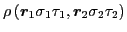, which depends on the space-spin-isospin nucleon coordinates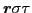, can be separated into four nonlocal spin-isospin densities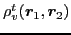as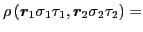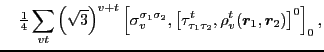(1)

where we sum over the spin-rank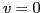and 1 (scalar and vector) and isospin-rank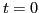and 1 (isoscalar and isovector) spherical tensors of the Pauli matrices,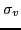and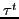, respectively. We denote vector (isovector) coupling by the standard square brackets with subscripts (superscripts) giving the values of the total spin (isospin).

For a local interaction, the energy corresponding to the direct (Hartree) term depends on the local densities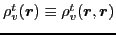in Eq. (1) as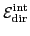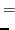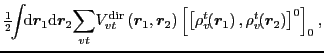(2)

where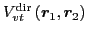denote the local direct two-body potentials. As discussed in Ref. , for short-range interactions and sufficiently smooth local densities one can employ simple Taylor expansions of densities to obtain the energy density in the form of a gradient expansion. As in Ref. , we use the spherical tensor representation of derivatives, which allows for the most economical classification of terms in the expansions.

Introducing the standard average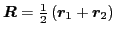and relative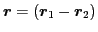coordinates, we have the following expansion of the local densities,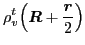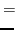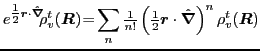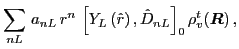(3)

where, for clarity, the spin and isospin projection quantum numbers,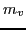and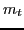, are not shown,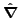denotes the gradient operator with respect to variable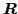,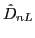denote the spherical-tensor derivatives of order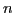and rank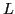,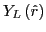are the standard spherical harmonics, and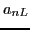are simple numerical constants resulting from the recoupling of the Cartesianth order derivatives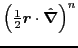into the spherical-tensor forms .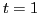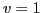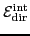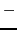12294.769 0 373.314 0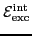594.992 0.62932.337 0.215

Expanded in this way, and after a partial integration and recoupling needed to make all derivative operators act on the same density, the direct interaction energy of Eq. (2) can be written as a gradient expansion,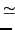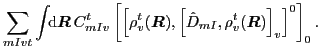(4)

In this final form, the coupling constants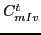are numbers obtained as moments of the direct potentials up to the order of the expansion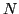. This order is defined by keeping all terms in the product that fulfill the condition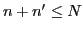, whereand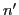denote the orders in the expansions of the two densities [compare with Eq. (3)].

To test the quality and convergence properties of this expansion, we generated a set of realistic test densities for doubly magic nuclei by solving the self-consistent equations for the nuclear SLy4 functional . For these test densities, the sums in Eq. (4) were evaluated to different orders by calculating the higher-order derivatives of densities needed using the program HOSPHE (v1.00) . The coupling constants in Eq. (4) were calculated from moments of the finite-range part of the Gogny D1S interaction . Then for the same set of test densities, the exact direct and exchange energies of the Gogny interaction were determined using the capability of treating Gaussian interactions with the code HFODD (v2.45h) .

As an example, in Table 1 we show the exact energies calculated in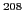Pb for the four spin-isospin channels. In the ground states of even-even nuclei, the vector terms of the direct energies vanish due to the conserved time-reversal symmetry, and thus our calculations here only test the scalar terms of the expansion in Eq. (4). In Fig. 1 we show percentage deviations between the gradient approximations and the exact results. It is extremely gratifying to see that in light nuclei the sixth-order expansion allows for reaching the precision of 0.1-0.01% or 0.1MeV. Even more important, in each higher order the precision increases by a large factor, which is characteristic to a rapid power-law convergence. One also sees that in heavier, isospin-unsaturated nuclei the precision slightly deteriorates, probably owing to a slower convergence for the isovector densities, which fluctuate more than the isoscalar ones.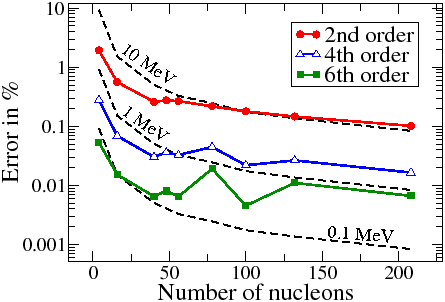One can, of course, quite easily calculate the direct terms exactly, but still it is interesting to see that a few of the lowest-order coupling constants appearing in the expansion are able to capture the essential physics contained in the nuclear finite-range force. For the exchange (Fock) terms whereto we turn now, the situation is significantly different, because here the expansion into quasi-local densities dramatically speeds up the calculations.

In order to deal with the exchange term, we propose a new approximation, which we call a damped Taylor (DT) expansion, and we define it in the following way: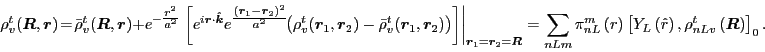(5)

The Taylor expansion of the term in the square brackets is obtained by using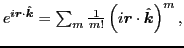where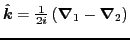is the standard relative-momentum operator. The quasi-local densities, are defined as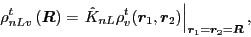(6)

where the higher-order spherical-tensor derivative operators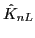are built from the first-order operators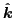. Because of the simple form of the expansion, explicit formulas for functions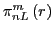can be derived and will be presented elsewhere .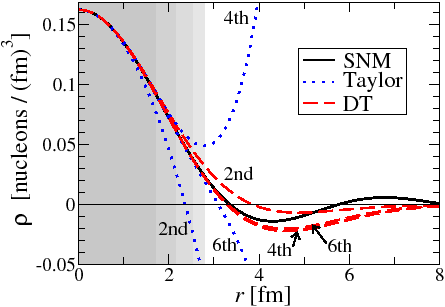The DT expansion has the potential to be more accurate than the original Negele-Vautherin (NV) expansion  because it avoids the approximation of angular averaging the density matrix in the nonlocal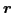direction. It is generated around a model density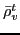and is also damped by a Gaussian factor with width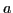. In the limit of vanishing model density and infinite damping distance, the DT becomes an ordinary Taylor expansion. This Taylor expansion applied to the uniform system of symmetric nuclear matter (SNM) is illustrated in Fig. 2. It works well in a region around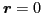but diverges for larger relative distances. These bad asymptotics can however be cured by using a finite damping width, as also demonstrated in Fig. 2. By employing the condition that it should work equally well in SNM for all values of Fermi momentum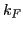, we estimate this width as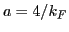. Assuming that the densities of real nuclei have similar behavior, we retain this estimate ofin the calculations presented below.

The model density can be chosen in different ways by the requirement that the truncated expansions must become exact in certain limits. The model used in the following leads to exact values in SNM, and is obtained by assuming that the density in each pointhas the SNM nonlocal dependence,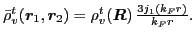For large relative distances, when the expansion around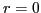can no longer be trusted, the bracketed term in Eq. (5) is damped to zero and the asymptotic behavior is then given entirely by this model part. In this way the parameterinterpolates between placing trust in the model part or in the expansion part, and the optimal balance is determined by the range in which the expansion converges, as estimated above.

By inserting the DT expansion of Eq. (5) into the expression for the exchange energy, we obtain the quasi-local expansion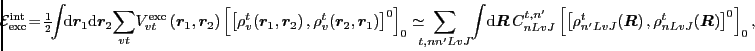(7)

where the quasi-local densities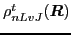, which were introduced in Ref. , are equal to those of Eq. (6) with the angular momentaand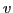coupled to the total angular momentum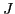. The coupling-constants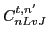are given by the integrals of the local exchange two-body potentials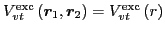and functionsof Eq. (5). They depend onthrough the model density and damping width, which in the standard local density approximation leads to a density dependence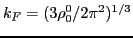.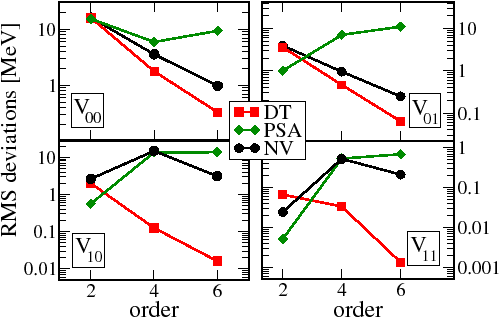In Fig. 3 we show results obtained with the DT expansion compared to the exact values, similar to those listed in Table 1. The comparison is made by considering the RMS deviations between the exact and approximate exchange energies, and is also shown for the NV  and phase-space averaging (PSA)  expansions. As seen in this figure, the NV Bessel-function expansion used in the scalar channels works very well, whereas the alternative method used for the vector channels [see Eq. (4.23) in Ref. ] does not improve when evaluated beyond the second order.

We employ the recently proposed PSA method in the INM-DME version  and generalize it to higher orders by incorporating the model density in the same way as in Eq. (5). Other modifications are probably also possible and could be studied as well. At second order, our prescription reproduces that proposed in Ref.  with the PSA performed over SNM. Fig. 3 shows that this is the best expansion at second order, where the total RMS deviation for the exchange energy is 14.09 MeV. When used for the vector part, the PSA expansion is similar to the NV expansion and also has problems when evaluated beyond second order.

As seen in Fig. 3, the DT expansion exhibits excellent convergence properties both for the vector and scalar parts, with every next order improving the results by large factors. Note that in the vector channels, the exact exchange energies of Table 1 are quite small, so at second order their relative description is poor. In contrast, at higher orders their description becomes rather accurate. The results obtained for the DT expansion are not so sensitive to the value of the damping width, and values in the range of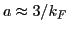-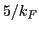all lead to improvements over the NV expansion.

The total RMS deviations obtained in the DT case for the exchange energies at fourth and sixth orders are 1.54 and 0.36MeV, respectively . Calculations using a fixed value of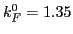fm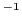give results that are not significantly different . They lead to substantial simplifications, because then the density dependencies of coupling constants can be neglected.

In summary, we presented the first analysis of density-matrix expansions extended beyond second order, with derivatives of densities included up to sixth order. We also proposed a new type of expansion that has excellent convergence properties. The results demonstrate that its possible to construct expansions into quasi-local density functionals that converge for the description of low-energy nuclear observables. This also facilitates the difficult adjustments of phenomenological higher-order nuclear functionals by providing inital guesses for free parameters as well as a method to derive them.

We thank Johan Grönqvist for useful discussions. This work is supported in part by the Academy of Finland and the University of Jyväskylä within the FIDIPRO programme, and by the Polish Ministry of Science under Contract No. N N202328234.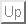Next: Bibliography
Jacek Dobaczewski 2010-07-28